##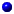Distance-dependent knowledge-based mean force potential

###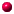Atom type definitions

ANOLEA uses a mean force potential at the atomic level, which is based on a particular definition of atom types. Forty different atom types are defined for all the heavy atoms of the 20 existant amino acids. In a strict physico-chemical point of view, all the atoms with different environments, connectivities and chemical nature, would be different. In the 20 amino acids the total number of heavy atoms is 167 and the number of non-equivalent heavy atoms is 98. We defined a total number of 40 different atom types for all the heavy atoms of the 20 amino acids (see figure below). The atom type definition is based on its connectivity, chemical nature and location level (side chain or backbone). For example, one type of heavy atom is the carbon of a methyl group bonded to a carbon with sp3 hybridization. This type represents the beta carbon of alanine side chain, the gamma1 and gamma2 carbons of valine, the delta1 and delta2 carbons of leucine, etc.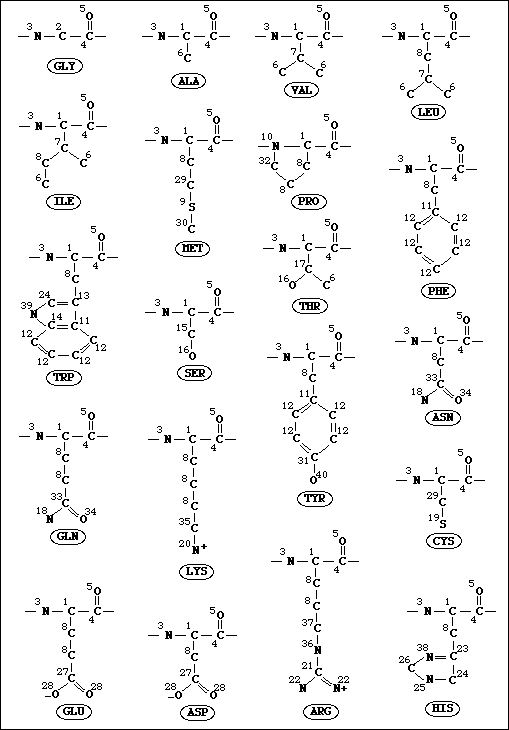###Mean force potential derivation

This mean force potential was calculated to evaluate the non-local environment of each atom in a protein molecule. The non-local environment of any given atom is defined as all the heavy atoms that are included within a 7 Ĺ radius sphere centered on the given atom, and that belong to amino acids that are more distant than 11 residues in the same chain or that belong to another chain. This definition is based on the observation of the energy curves of a previous mean force potential that included local and non-local interactions, where sequence separations higher than 11 do not affect the shape of the energy functions for the different pairwise interactions. As an example, one energy curve of this previous mean force potential is shown in the figure below.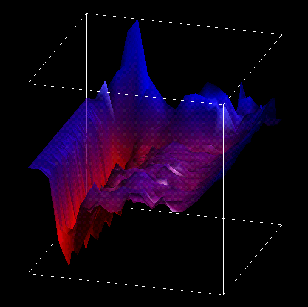The current potential has been derived using 35 different classes of distance (l), ranging from 0 to 7 Å by steps of 0.2 Å . All pairwise ocurrences out of this range were excluded. We considered all the pairs of atoms ocurring within an Euclidean distance of 7 Å , that belong to amino acids that are farther than 11 residues in the chain or that belong to different chains (if you want to see a scheme of this click here). A sequence separation of 2 means that two amino acids are adjacent in the sequence.

The potential was obtained from a database of 147 non-redundant protein chains. The calculation was performed over all these chains. In the case of multimers, all the atom contacts with the other chains were considered.

We have compiled each pairwise contact symmetrically, obtaining 820 (40x41/2) different atom pairwise distributions. The calculation of pairwise pseudo-energy terms has been carried out as described by Sippl (1990). The following expression was used: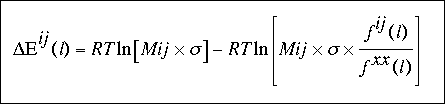where Mij is the total number of observations for the atomic pair ij and corresponds to: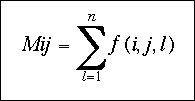sigma represents the weight given to each observation. We have used sigma = 1/50 as proposed by Sippl (1990), such that for 50 observations, Fij (l) and Fxx (l) have the same weight in the calculation of Eij (l).

Fij (l) is the relative frequency of ocurrence of the atomic pair ij in the class of distance l and corresponds to: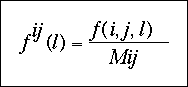Fxx(l) is the relative frequency of ocurrence of all the atomic pairs in the class of distance l and can be expressed as: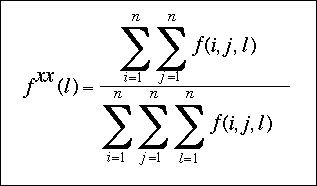The temperature was set to 293K, so that RT is equal to 0.582 kcal/mol.

##Bibliography

WebmasterGo back to ANOLEA home page